## Profiling Arduino Code

William F. Dudley
2014 January 5

Sometimes it's helpful to see where the cpu is "spending it's time" in your program (sketch). In a proper (big bucks) development environment, the engineer has access to profiling hardware. This hardware samples the program counter of the target on a regular basis (say, every millisecond) and then counts each time the program counter value lands in a "bin". Each bin represents a range of addresses.

For example, you might divide your program address space into 100 bins, and then run the profiler. The output is a histogram showing the number of times the sampled program counter value was within the range defined for each bin.

On the Arduino, we don't have the luxury of a proper emulator, and we don't really have enough RAM to divide our program space into lots of bins and have a counter for each bin. In addition, we don't, without a lot of gcc-fu, have a way to get a listing that maps addresses in program space to lines in our C program (sketch).

My solution is to instead manually define a "bin" for arbitrary chunks of code. One good division is to create a "bin" for each subroutine in your sketch. When you run the profiler, you will then get a histogram showing the relative time spent in each subroutine in your sketch. If a subroutine is long and has several complex sections, you can always subdivide that subroutine into multiple "bins" so you can get finer detail in the histogram.

To use the profiler, you must create (or modify) a timer interrupt service routine that runs at some reasonable rate; I suggest 1 kilohertz. This ISR does the sampling of what "bin" your code is executing every time it runs (once a millisecond.) Then you sprinkle PF(n) macros around your sketch, where "n" is a number between 1 and the number of bins you decide on (minus 2). If you want a histogram with 10 bins, that's 20 bytes of storage (bins are unsigned ints), and the PF() macros that are legal are between PF(1) and PF(8), inclusive. PF(9) is reserved for time spent in mydelay() which is just a wrapper around delay(). There is a default value of 30 bins but that number can be changed with a #define MAXPROF n.

When you call delay(), you're "wasting" cpu cycles. If you call mydelay() instead, you're still wasting cycles, but the profiler will count that time for you and put it in the ultimate bin. (When you set MAXPROF to 8, then time spent in mydelay() will end up in bin "7".) Time spent in the profiler is put in bin "0".

Because this runs for 1 minute, and the interrupt is running at 1KHz, the maximum number of counts that the bins will see is 60000. (I get a hair over 60000; this is because the "every 1 minute" printing of the results is not exact.) At any rate, 60000 conveniently fits in an unsigned int. So if you change the sample rate from 1KHz, or change the sample time from 1 minute, you may have to change the bins from unsigned ints to longs, which will double the RAM requirement and also add a small bit of overhead due to the "long arithmetic" taking more time.

Here is a simple example program that shows how to use my profiler on your code. You will notice that the two "make work" functions have __attribute__ ((noinline)) in their definition. These attributes were the end result of several hours of research, which started when my profiler "didn't work" on this simple example code. If you're curious, read about that odyssey here.

```// example code for Profiling Arduino Code
// http://www.dudley.nu/arduino_profiling
// William F. Dudley Jr.
// 2014 January 5

#include <avr/interrupt.h>
#include <avr/io.h>
#include <HardwareSerial.h>

// this can be used to turn profiling on and off
#define PROFILING 1
// this needs to be true in at least ONE .c, .cpp, or .ino file in your sketch
#define PROFILING_MAIN 1
// override the number of bins
#define MAXPROF 8
#include "profiling.h"

// some handy macros for printing debugging values
#define DL(x) Serial.print(x)
#define DLn(x) Serial.println(x)
#define DV(m, v) do{Serial.print(m);Serial.print(v);Serial.print(" ");}while(0)
#define DVn(m, v) do{Serial.print(m);Serial.println(v);}while(0)

// more handy macros but unused in this example
#define InterruptOff  do{TIMSK2 &= ~(1<<TOIE2)}while(0)
#define InterruptOn  do{TIMSK2 |= (1<<TOIE2)}while(0)

// stuff used for time keeping in our ISR
volatile unsigned int int_counter;
volatile unsigned char seconds, minutes;
unsigned int tcnt2; // used to store timer value

// Arduino runs at 16 Mhz, so we have 1000 overflows per second...
// this ISR will get hit once a millisecond
ISR(TIMER2_OVF_vect) {

int_counter++;
if (int_counter == 1000) {
seconds++;
int_counter = 0;
if(seconds == 60) {
seconds = 0;
minutes++;
}
}
#if PROFILING
prof_array[prof_line]++;
#endif
TCNT2 = tcnt2;  // reset the timer for next time
}

// Timer setup code borrowed from Sebastian Wallin
// http://popdevelop.com/2010/04/mastering-timer-interrupts-on-the-arduino/
// further borrowed from: http://www.desert-home.com/p/super-thermostat.html
void setupTimer (void) {
//Timer2 Settings:  Timer Prescaler /1024
// First disable the timer overflow interrupt while we're configuring
TIMSK2 &= ~(1<<TOIE2);
// Configure timer2 in normal mode (pure counting, no PWM etc.)
TCCR2A &= ~((1<<WGM21) | (1<<WGM20));
// Select clock source: internal I/O clock
ASSR &= ~(1<<AS2);
// Disable Compare Match A interrupt enable (only want overflow)
TIMSK2 &= ~(1<<OCIE2A);

// Now configure the prescaler to CPU clock divided by 128
TCCR2B |= (1<<CS22)  | (1<<CS20); // Set bits
TCCR2B &= ~(1<<CS21);             // Clear bit

/* We need to calculate a proper value to load the timer counter.
* The following loads the value 131 into the Timer 2 counter register
* The math behind this is:
* (CPU frequency) / (prescaler value) = 125000 Hz = 8us.
* (desired period) / 8us = 125.
* MAX(uint8) - 125 = 131;
*/
/* Save value globally for later reload in ISR */
tcnt2 = 131;

/* Finally load end enable the timer */
TCNT2 = tcnt2;
TIMSK2 |= (1<<TOIE2);
sei();
}

volatile long long_waste;

// because this has "PF(2)", when the processor is executing here, it
// will cause the value in bin "2" to increase.
// "__attribute__ ((noinline))" needed to prevent inlining of these
// trivial functions.  The inlining by the compiler broke the profiling.
void __attribute__ ((noinline)) big_cpu_fn_1 (void) {
long lo;
PF(2);
for(lo = 1L ; lo < 8600L ; lo++) {
long_waste += lo;
}
// DVn("1 lw ", long_waste);
}

// because this has "PF(3)", when the processor is executing here, it
// will cause the value in bin "3" to increase.
// "__attribute__ ((noinline))" needed to prevent inlining of these
// trivial functions.  The inlining by the compiler broke the profiling.
void __attribute__ ((noinline)) big_cpu_fn_2 (void) {
long lo;
PF(3);
for(lo = 1L ; lo < 29900L ; lo++) {
long_waste -= lo;
}
// DVn("2 lw ", long_waste);
}

void setup (void) {
#if PROFILING
PF(0);
prof_has_dumped = 0;
clear_profiling_data();
#endif
Serial.begin(9600);
Serial.println("setup()");

int_counter = 0;
seconds = 0;
minutes = 0;

Serial.println("setupTimer()");
setupTimer();
pinMode(1, OUTPUT);
}

// the stuff inside "#if PROFILING" is necessary for the profiler; that's
// the results are dumped to the debug window, once a minute.
void loop (void) {
unsigned char op;

PF(1);
big_cpu_fn_1();	// goes in bin 2
big_cpu_fn_2();	// goes in bin 3
mydelay(20);	// goes in bin 7
PF(1);	// rest of this should go in profiling bin 1
op ^= 1;
digitalWrite(1, op & 1);	// toggle a pin so we can see loop rate
if(int_counter < 110) { DVn("sec ", seconds); }
#if PROFILING
if(seconds % 60 == 3 && !prof_has_dumped) {
dump_profiling_data();	// also clears profiling data
}
if(seconds % 60 == 4 && prof_has_dumped) {
prof_has_dumped = 0;
}
#endif
}
```

Here's the include file that supplies the rest of the profiler:
```#ifndef PROFILING_H

#if PROFILING

#ifndef MAXPROF
#define MAXPROF 30
#endif

#define PF(n) do{prof_line=n;}while(0)
#define mydelay(v) do{PF(MAXPROF-1);delay(v);}while(0)

#if PROFILING_MAIN
#define EXTERN
#else
#define EXTERN extern
#endif

EXTERN volatile unsigned int prof_array[MAXPROF];
EXTERN volatile unsigned int prof_line;
EXTERN volatile unsigned char prof_has_dumped;

#if PROFILING_MAIN

#define _DL(x) Serial.print(x)
#define _DLn(x) Serial.println(x)

void clear_profiling_data (void) {
unsigned char c;
for(c = 0 ; c < MAXPROF ; c++) {
prof_array[c] = 0;
}
}

void dump_profiling_data(void) {
unsigned char c;
prof_has_dumped = 1;
PF(0);
for(c = 0 ; c < MAXPROF ; c++) {
_DL((int)c);
_DL(" ");
_DLn((unsigned int)prof_array[c]);
prof_array[c] = 0;
}
}
#endif

#else
#define PF(n)
#define mydelay(v) delay(v)
#endif

#define PROFILING_H 1
#endif
```

Here's a portion of the sample output from the example program. It prints the time in seconds (mod 60), and between second 3 and second 4, it dumps the values in the bins, and then clears the bin counters.

You can see that a little more time is spent in mydelay() than in big_cpu_fn_1(), and almost 4 times as much cpu is spent in big_cpu_fn_2(). A tiny amount of overhead is spent in the profiler itself (bin 0).

In the output below, the lines starting with "sec" are just timestamps showing elapsed time. The lines starting with an integer are the bin data; first number is bin number, second number is number of hits in that bin during the 1 minute sample time. Specifically, bin 2 represents time spent in big_cpu_fn_1(), and bin 3 represents time spent in big_cpu_fn_2(). Time spent in mydelay() is shown as bin 7.

```. . .
sec 2
sec 3
0 3
1 0
2 10738
3 37301
4 0
5 0
6 0
7 11991
sec 4
sec 5
. . .
```

To extract it: tar xzvf prof_eg.tgz

Here's a zip file containing the code shown on this page: prof_eg.zip.

William F. Dudley
2014 January 5

## FAQ

1. Why not use the program counter instead of arbitrary numbers and map addresses to listing locations?

I thought that having to run another process (avr-objdump) outside of the IDE in order to map addresses to code locations was more complexity than I wanted in the process. If the IDE automated that it would be a different story. Since I've given the code away, you're free to build this anyway you like, and I hope you'll post your code when you do.

2. Why not use symbolic names for the bins?

It's certainly possible to make symbolic names for the bins. I chose not to because I wanted this to be simple to code. One could do something like this:

```char *pf_symbols = { "overhead", "big fn 1", "big fn 2",
"n.a.", "n.a.", "n.a.", "n.a.", "delay" };
#define BIGFN1 2
#define BIGFN2 3
#define DELAY  7
. . .
PF(BIGFN1);
. . .
PF(BIGFN2);
. . .
// new version of dump_profiling_data():
void dump_profiling_data(void) {
unsigned char c;
prof_has_dumped = 1;
PF(0);
for(c = 0 ; c < MAXPROF ; c++) {
_DL(pf_symbols[c]);
_DL(" ");
_DLn((unsigned int)prof_array[c]);
prof_array[c] = 0;
}
}
```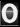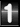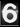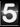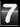Views